Next: Time-resolved spectra and non-linear Up: Modeling time-resolved spectra Previous: Modeling time-resolved spectra

# Introduction

ZZ Ceti stars are variable DA white dwarfs (WDs) with effective temperatures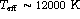. Their amplitudes range from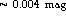to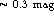. The pulsations are non-radial g modes with periods of the order of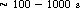. A well-known property of g modes is that radius variations are negligible because of the high surface gravity (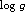) of WDs (Robinson et al. 1982; hereafter RKN). Therefore, one can think of these oscillations as temperature waves.

Recently, Brassard et al. (1995; hereafter BFW) calculated spectra semi-analytically for temperature distributions across the surface expressed as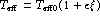whereis a sum of spherical harmonics. The aim of this analysis was to investigate non-linear effects that occur since the specific intensity I is not a linear function of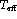. We have developed a program that calculates the time-variable spectrum by integrating I over the visible disk of the WD for a distribution ofacross the WD surface. For now, we use foran expression similar to that of BFW. However, we can adapt our program to non-linear variations of, which were suggested by Brickhill (1992) and could be caused by the response of the convective flux to linear pressure perturbations in deeper layers of these stars. We interpolate I from a grid containing I as a function of,,,and different parametrizations of the mixing length theory (MLT). The grid was calculated with a stellar atmospheres code.

Horst Vaeth
Thu Sep 26 10:49:14 MET DST 1996